TitleCollege Algebra
Answer/Discussion to Practice Problems
Tutorial 54D: Geometric Sequences and
SeriesAnswer/Discussion to 1aSince a geometric sequence is a sequence, you find the terms exactly the same way that you do a sequence.   n is our term number and we plug the term number into the function to find the value of the term. If you need a review on sequences, feel free to go to Tutorial 54A: Sequences. Lets see what we get for our first five terms: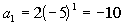*n = 1*n = 2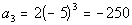*n = 3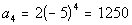*n = 4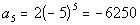*n = 5

 What would be the common ratio for this problem? If you said -5 you are correct!  Note that you would have to multiply (-10)(-5) = 50, (50)(-5) = -250, (-250)(5) = 1250, and (1250)(-5) = -6250.   It has to be consistent throughout the sequence.  Also note that the base that is being raised to a power is -5.Answer/Discussion to 1b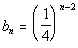Since a geometric sequence is a sequence, you find the terms exactly the same way that you do a sequence.   n is our term number and we plug the term number into the function to find the value of the term. If you need a review on sequences, feel free to go to Tutorial 54A: Sequences. Lets see what we get for our first five terms: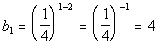*n = 1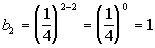*n = 2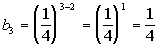*n = 3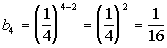*n = 4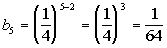*n = 5

 What would be the common ratio for this problem? If you said 1/4 you are correct!  Note that you would have to multiply by 1/4 each time you go from one term to the next: (4)(1/4) = 1, (1)(1/4) = 1/4,  (1/4)(1/4) = 1/16, and (1/16)(1/4)=1/64.   It has to be consistent throughout the sequence.  Also note that the base that is being raised to a power is 1/4.Answer/Discussion to 2a 125, 25, 5, 1, ...

 We will use the nth term formula for a geometric sequence,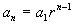to help us with this problem. Basically we need to find two things: the first term of the sequence,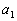and  the common ratio, r. What is, the first term? If you said 125, give yourself a high five.  The first term of this sequence is 125. What is r, the common ratio? If you said 1/5, you are right!! Note that you would have to multiply 1/5 each time you go from one term to the next: (125)(1/5) = 25, (25)(1/5) = 5, and (5)(1/5) = 1.   It has to be consistent throughout the sequence. Putting in 125 forand 1/5 for r we get: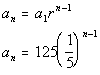Answer/Discussion to 2b 4, -12, 36, -108, ...

 We will use the nth term formula for a geometric sequence,to help us with this problem. Basically we need to find two things: the first term of the sequence,and  the common ratio, r. What is, the first term? If you said 4, give yourself a high five.  The first term of this sequence is 4. What is r, the common ratio? If you said -3, you are right!! Note that you would have to multiply -3 each time you go from one term to the next: (4)(-3) = -12, (-12)(-3) = 36, and (36)(-3) = -108.   It has to be consistent throughout the sequence. Putting in 4 forand -3 for r we get: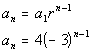Answer/Discussion to 3a 2 + 14 + 98 + 686 + 4802 + 33614 + 235298

 We will use the formula for the sum of the first n terms of geometric sequence,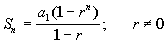, to help us with this problem. Basically we need to find three things: the first term of the sequence, the common ratio, and how many terms of the sequence we are adding in the series. What is, the first term? If you said 2 you are right! What is r, the common ratio? If you said 7, give yourself a pat on the back. Note that you would have to multiply -2 each time you go from one term to the next: (2)(7) = 14, (14)(7) = 98, (98)(7) = 686, (686)(7) = 4802, (4802)(7) = 33614, and (33614)(7) = 235298.   It has to be consistent throughout the sequence. How many terms are we summing up? If you said 7, you are correct. Putting in 2 for the first term, 7 for the common ratio, and 7 for n, we get: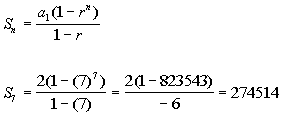Answer/Discussion to 3b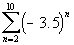We will use the formula for the sum of the first n terms of geometric sequence,, to help us with this problem. Basically we need to find three things: the first term of the sequence, the common ratio, and how many terms of the sequence we are adding in the series. What is, the first term? If you said 12.25 you are right!   Since this summation starts at 2, you need to plug in 2 into the given formula: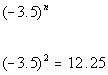What is r, the common ratio? If you said -3.5, give yourself a pat on the back.  Note that -3.5 is the number that is being raised to the exponent.  So each time the number goes up on the exponent, in essence you are multiplying it by -3.5. How many terms are we summing up? If you said 9, you are correct.  If you start at 2 and go all the way to 10, there will be 9 terms. Putting in 12.25 for the first term, -3.5 for the common ratio, and 9 for n, we get: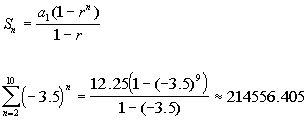Answer/Discussion to 4a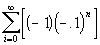We will use the formula for the sum of infinite geometric sequence,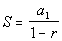,  to help us with this problem. Basically we need to find two things: the first term of the sequence and the common ratio. What is the first term,? If you said -1 you are right!   Since this summation starts at 0, you need to plug in 0 into the given formula: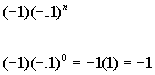What is the common ratio, r? If you said -.1, give yourself a pat on the back. Note that -.1 is the number that is being raised to the exponent.  So each time the number goes up on the exponent, in essence you are multiplying it by -.1. Putting in -1 for the first term and -.1 for the common ratio we get: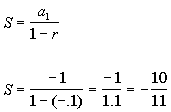Answer/Discussion to 4bWe will use the formula for the sum of infinite geometric sequence,,  to help us with this problem. Basically we need to find two things: the first term of the sequence and the common ratio. What is the first term,? If you said 1 you are right! What is the common ratio, r? If you said -3/2, give yourself a pat on the back. Note that you would have to multiply -3/2 each time you go from one term to the next: (1)(-3/2) = -3/2, (-3/2)(-3/2) = 9/4, (9/4)(-3/2) = -27/8.   It has to be consistent throughout the sequence. Since the geometric ratio is -3/2 and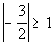, there is no sum.

Last revised on May 16, 2011 by Kim Seward.
All contents copyright (C) 2002 - 2011, WTAMU and Kim Seward. All rights reserved.